1. 2. 3.

## 不是那两个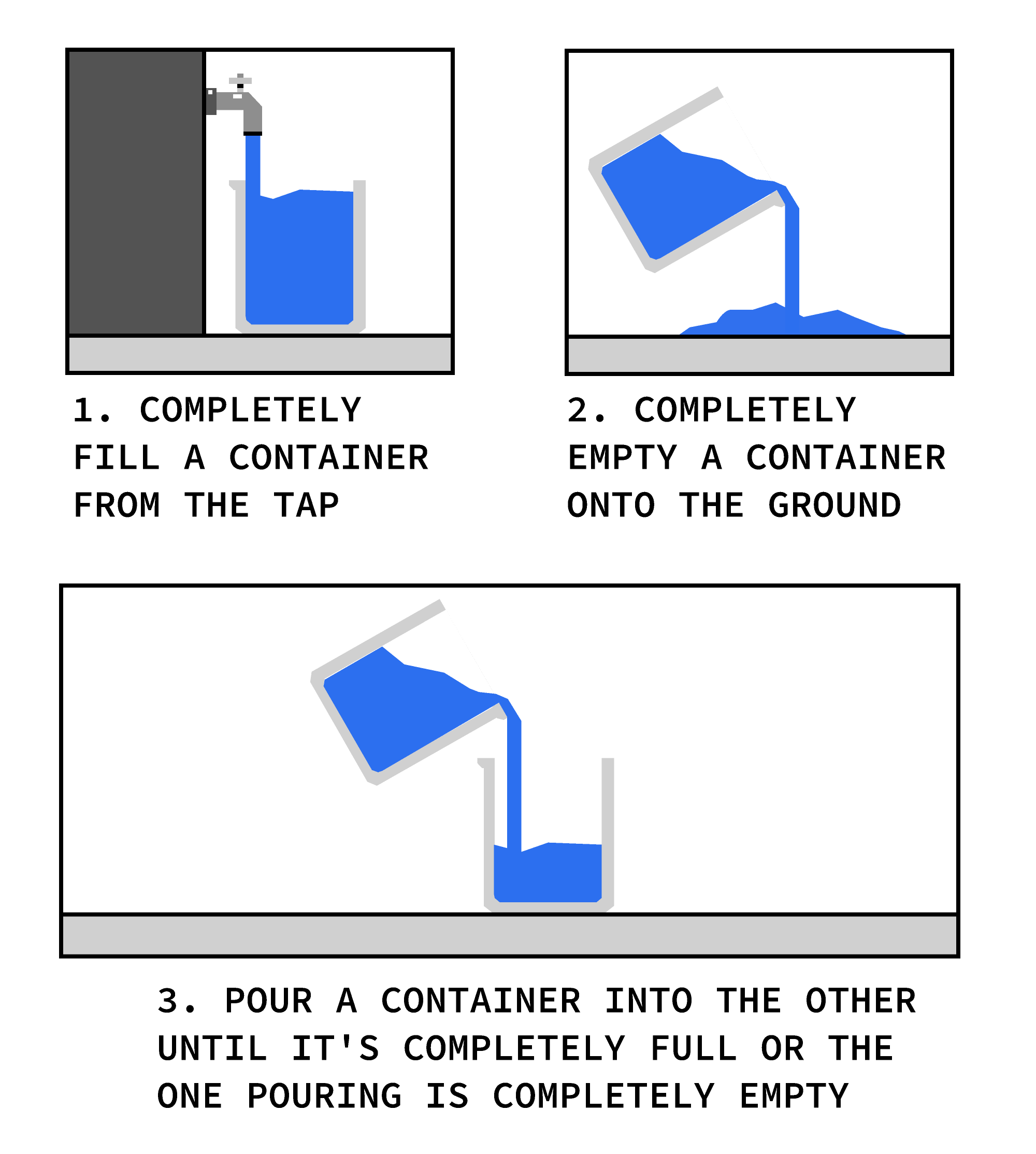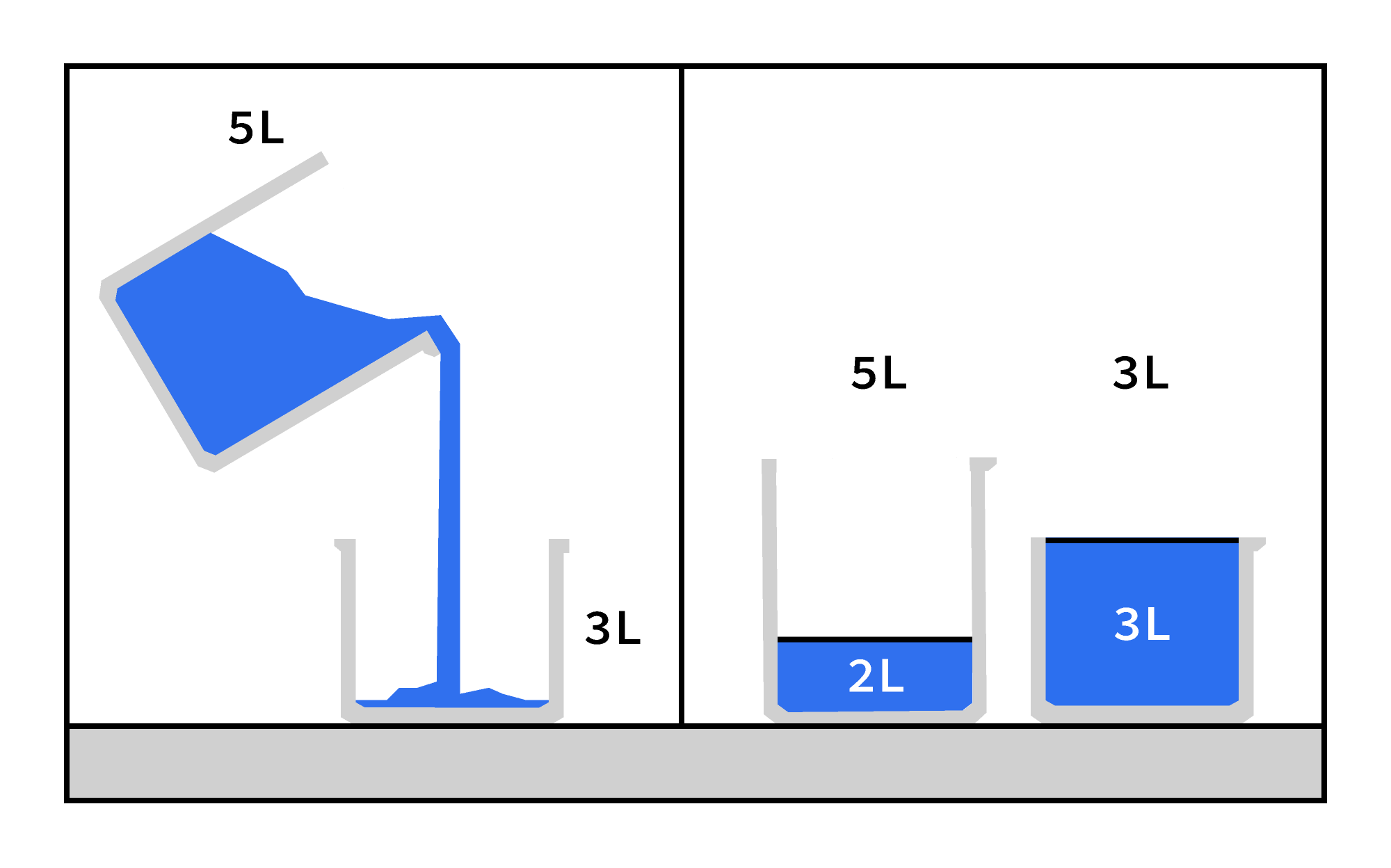1. 填满 $3.$-升瓶。
2. 清空 $3.$-把一公升的瓶子装进桶里 $5.$-升瓶。
3. 重新装满 $3.$-升瓶。
4. $3.$-把一公升的瓶子装进桶里 $5.$-升瓶子，直到大瓶子装满。 $\\[-0.8em]$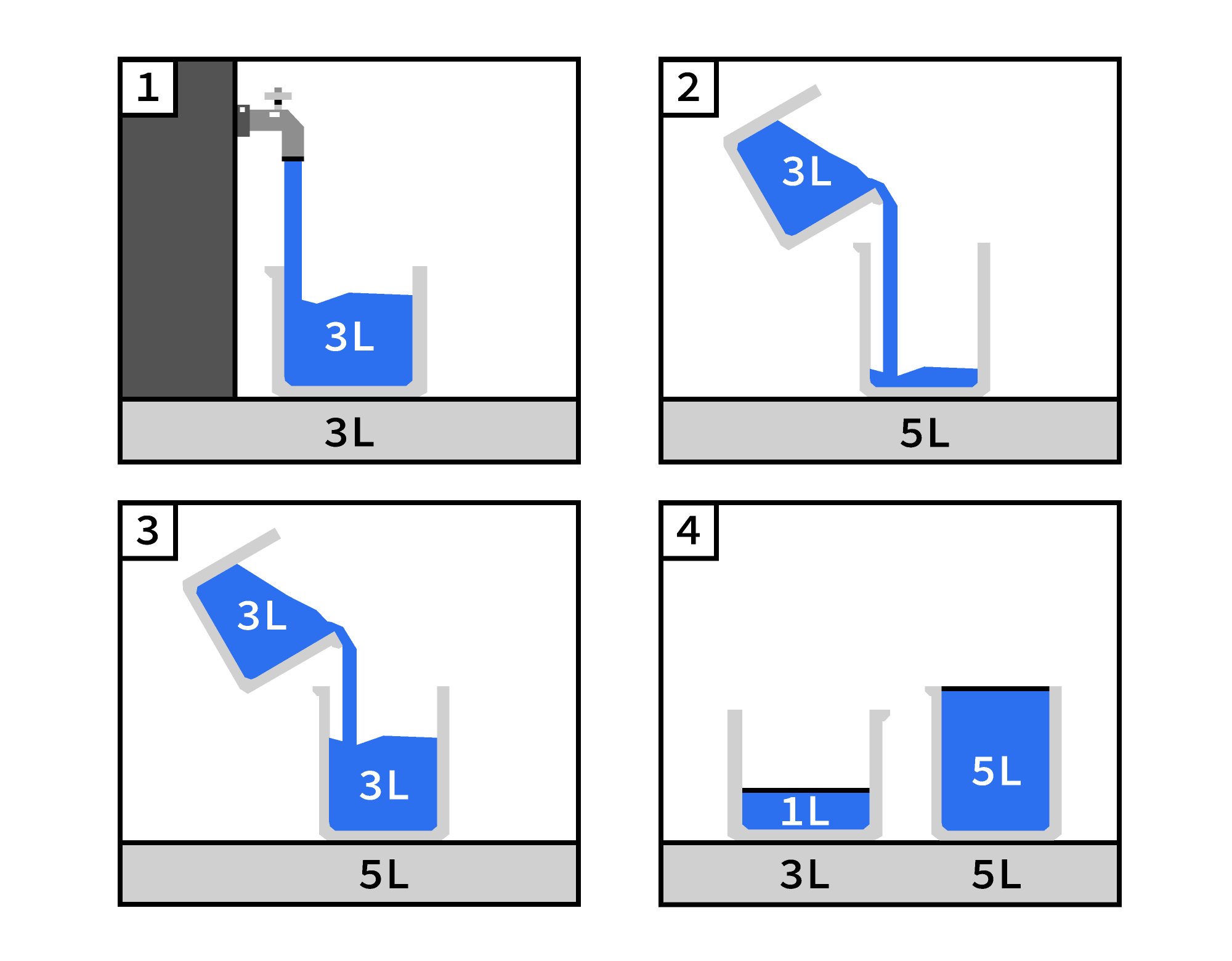# 今天的挑战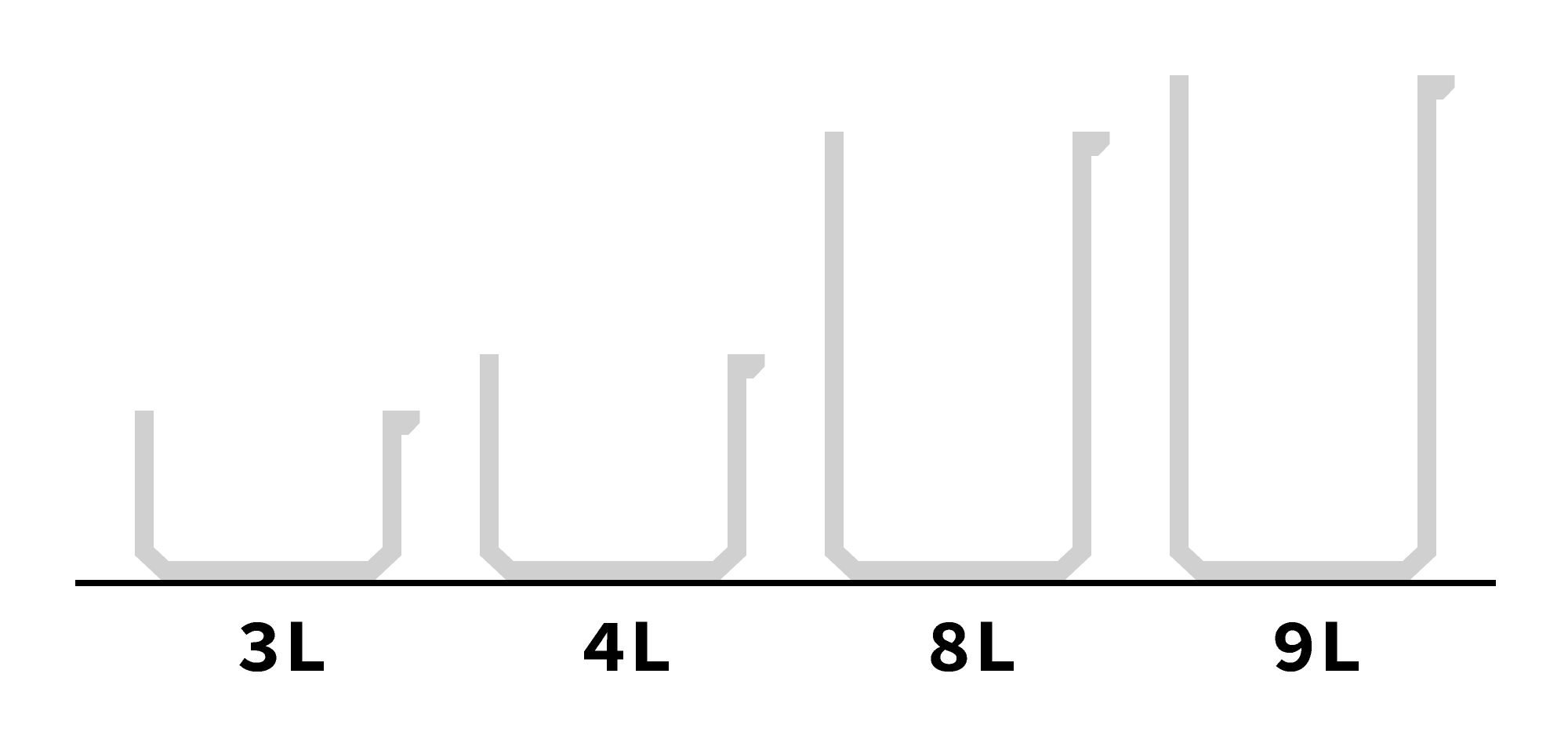• 从流中完全填充容器。
• 将容器完全倒在地上。
• 将一个容器倒入另一个容器，直到容器完全装满或一个容器完全空。 $\\[-0.7em]$

$\\[-0.5em]$如果马文只能将其中两个容器带到溪流中，以下哪种组合会让他精确地测量 $1.$公升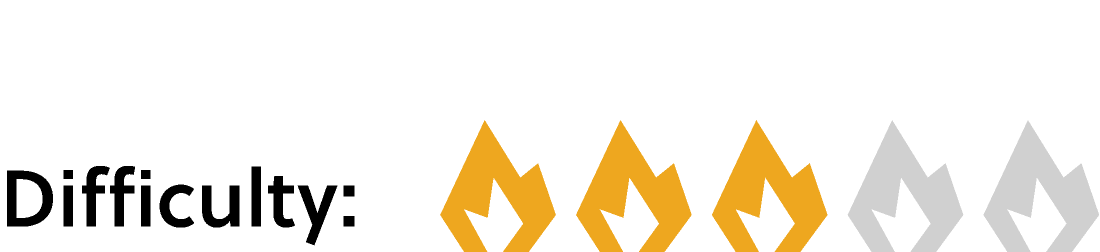×